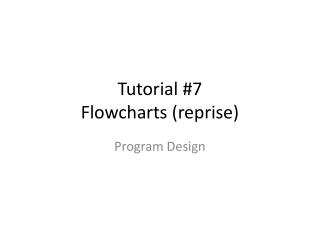# Tutorial #7 Flowcharts (reprise) - PowerPoint PPT PresentationDownload PresentationTutorial #7 Flowcharts (reprise)

Tutorial #7 Flowcharts (reprise)Download Presentation## Tutorial #7 Flowcharts (reprise)

- - - - - - - - - - - - - - - - - - - - - - - - - - - E N D - - - - - - - - - - - - - - - - - - - - - - - - - - -
##### Presentation Transcript

1. Tutorial #7Flowcharts (reprise) Program Design

2. Introduction • We mentioned it already, that if we thing of an analyst as being analogous to an architect, and a developer as being analogous to a builder, then the most important thing we can do as analysts is to explain our designs to the developers in a simple and clear way. • How do architects do this?

3. Symbols

4. Symbols

5. Symbols Decision Terminal Input/Output Operation Connector Module Process

6. Flowcharts • So we start the program as: START

7. Flowcharts • Our program will finish with the following: END

8. SEQUENCE

9. Flowcharts • When we write programs, we assume that the computer executes the program starting at the beginning and working its way to the end. • This is a basic assumption of all algorithm design.

10. Flowcharts • When we write programs, we assume that the computer executes the program starting at the beginning and working its way to the end. • This is a basic assumption of all algorithm design. • We call this SEQUENCE.

11. START Statement1 Statement2 END

12. SELECTION

13. Flowcharts • What if we want to make a choice, for example, do we want to add sugar or not to the tea?

14. Flowcharts • What if we want to make a choice, for example, do we want to add sugar or not to the tea? • We call this SELECTION.

15. START Is Sugar required? Add Sugar Don’t Add Sugar No Yes END

16. ITERATION

17. Flowcharts • What if we need to tell the computer to keep doing something until some condition occurs?

18. Flowcharts • What if we need to tell the computer to keep doing something until some condition occurs? • Let’s say we wish to indicate that the you need to keep filling the kettle with water until it is full.

19. Flowcharts • What if we need to tell the computer to keep doing something until some condition occurs? • Let’s say we wish to indicate that the you need to keep filling the kettle with water until it is full. • We need a loop, or ITERATION.

20. START Keep Filling Kettle Kettle is not full? No Yes END

21. EXAMPLES

22. Flowcharts • So let’s say we want to express the following algorithm: • Read in a number and print it out.

23. START

24. START Read in A

25. START Read in A Print A

26. START Read in A Print A END

27. Flowcharts • So let’s say we want to express the following algorithm: • Read in a number and print it out double the number.

28. START

29. START Read in A

30. START Read in A Print A*2

31. START Read in A Print A*2 END

32. Or alternatively...

33. START

34. START Read in A

35. START Read in A B = A*2

36. START B = A * 2 can be read as “B gets the value of A multiplied by 2” Read in A B = A*2

37. START Read in A B = A*2 Print B

38. START Read in A B = A*2 Print B END

39. Flowcharts • So let’s say we want to express the following algorithm: • Read in a number, check if it is odd or even.

40. START

41. START Read in A

42. START Read in A Does A/2 give a remainder?

43. START Read in A Does A/2 give a remainder? Print “It’s Odd” Yes

44. START Read in A Does A/2 give a remainder? Print “It’s Odd” Print “It’s Even” No Yes

45. START Read in A Does A/2 give a remainder? Print “It’s Odd” Print “It’s Even” No Yes END

46. Flowcharts • So let’s say we want to express the following algorithm to print out the bigger of two numbers: • Read in two numbers, call them A and B. Is A is bigger than B, print out A, otherwise print out B.

47. START

48. START Read in A and B

49. START Read in A and B A>B?